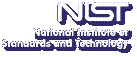Up# Sufficiency Class for Global (in Time) Solutions to the Three-Dimensional Navier-Stokes Equations

Tepper L. Gill
Howard University, Department of Electrical and Computer Engineering

Tuesday, February 6, 2007 15:00-16:00,
Building 101, Lecture Room D
Gaithersburg
Tuesday, February 6, 2007 13:00-14:00,
Room 4511
Boulder

Abstract: A well-known unsolved problem (in the classical theory of fluid mechanics) is to identify a set of initial velocities, which may depend on the viscosity, the body forces, and possibly the boundary of the fluid, that will allow global in time solutions to the three-dimensional Navier-Stokes equations. These equations describe the time evolution of the fluid velocity and pressure of an incompressible viscous homogeneous Newtonian fluid in terms of a given initial velocity and given external body forces. A related problem is to provide conditions under which we can be assured that the numerical approximation of these equations, used in a variety of fields from weather prediction to submarine design, has only one solution. In this talk, I will discuss an approach, based on additional physical insight, which allows us to prove that there exists a number u+ such that for all initial velocities in a ball of radius u+, the Navier-Stokes equations have unique global in time solutions and the numerical approximation of these equations has only one solution.

Presentation Slides: PDF (Slides), PDF (Global (in Time) Solutions to the 3D-Navier-Stokes Equations on R3), PDF (Sufficiency Class for Global (in Time) Solutions to the 3D-Navier-Stokes Equations), PDF (Sufficiency Class for Global (in Time) Solutions to the 3D-Navier-Stokes Equations in V)

Contact: F. Hunt

Note: Visitors from outside NIST must contact Robin Bickel; (301) 975-3668; at least 24 hours in advance.